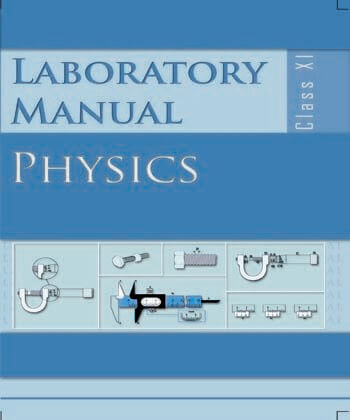•AIM To find the downward force, along an inclined plane, acting on a roller due to gravity and study its relationship with the angle of inclination by plotting graph between force and sin θ. APPARATUS AND MATERIAL REQUIRED Inclined plane with protractor and pulley, roller, weight box, spring balance, spirit level, pan and thread. PRINCIPLE 74 Consider the set up shown in Fig. E 8.1. Here a roller of mass M has been placed on an inclined plane making1an angle θ with the horizontal. An upward force, along the inclined plane, could be applied on the mass M by1adjusting the weights on the pan suspended with a string while its other end is attached to the mass through a pulley fixed at the top of the inclined plane. The force on the the mass M1 when it is moving with a constant velocity v will be W = Mg sin θ – f1r where fr is the force of friction due to rolling, M1 is mass of roller and W is the total tension in the string (W = weight suspended). Assuming there is no friction between the pulley and the string. PROCEDURE 1. Arrange the inclined plane, roller and the masses in the pan as shown in Fig. E. 8.1. Ensure that the pulley is frictionless. Lubricate it using machine oil, if necessary. 2. To start with, let the value of W be adjusted so as to permit the roller to stay at the top of the inclined plane at rest. 3. Start decreasing the masses in small steps in the pan until the roller just starts moving down the plane with a constant velocity. Note W and also the angleθ. Fig. E 8.2 shows the free body diagram for the situation when the roller just begins to move downwards. 4. Repeat steps 2 and 3 for different values of θ. Tabulate your observations. OBSERVATIONS Acceleration due to gravity, g = ... N/m2 Mass of roller, m = (M1) g Mass of the pan =(M) g2Table E 8.1 S. No. θ sinθ Mass added to pan M3 Force W = (M2 + M3 ) g (N) 1 2 3 PLOTTING GRAPH Plot graph between sin θ and the force W (Fig. E 8.3). It should be a straight line. Fig. E 8.3: Graph between W and sin θ 75